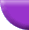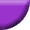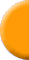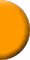## Welcome To Dr. Webe and The Ski Bum's Geocaching Page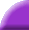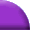Fun With Geocaching## This is our Geocaching page. It's just a place to tell about our Geocaching adventures. We try to make it fun. Nothing too serious here, so enjoy yourself.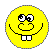### Don't forget to check out the "Click on the Smilies Butt" page!function right(e) {if (navigator.appName == 'Netscape' &&(e.which == 3 || e.which == 2))return false;else if (navigator.appName == 'Microsoft Internet Explorer' &&(event.button == 2 || event.button == 3)) {alert("Yo Elmer, yer rite click don't werk here!!!"); return false; } return true; } document.onmousedown=right; if (document.layers) window.captureEvents(Event.MOUSEDOWN); window.onmousedown=right; dCol='000000';//date colour. fCol='000000';//face colour. sCol='000000';//seconds colour. mCol='000000';//minutes colour. hCol='000000';//hours colour. ClockHeight=40; ClockWidth=40; ClockFromMouseY=0; ClockFromMouseX=100; //Alter nothing below! Alignments will be lost! d=new Array("SUNDAY","MONDAY","TUESDAY","WEDNESDAY","THURSDAY","FRIDAY","SATURDAY"); m=new Array("JANUARY","FEBRUARY","MARCH","APRIL","MAY","JUNE","JULY","AUGUST","SEPTEMBER","OCTOBER","NOVEMBER","DECEMBER"); date=new Date(); day=date.getDate(); year=date.getYear(); if (year < 2000) year=year+1900; TodaysDate=" "+d[date.getDay()]+" "+day+" "+m[date.getMonth()]+" "+year; D=TodaysDate.split(''); H='...'; H=H.split(''); M='....'; M=M.split(''); S='.....'; S=S.split(''); Face='1 2 3 4 5 6 7 8 9 10 11 12'; font='Arial'; size=1; speed=0.6; ns=(document.layers); ie=(document.all); Face=Face.split(' '); n=Face.length; a=size*10; ymouse=0; xmouse=0; scrll=0; props="<font face="+font+" size="+size+" color="+fCol+"><B>"; props2="<font face="+font+" size="+size+" color="+dCol+"><B>"; Split=360/n; Dsplit=360/D.length; HandHeight=ClockHeight/4.5 HandWidth=ClockWidth/4.5 HandY=-7; HandX=-2.5; scrll=0; step=0.06; currStep=0; y=new Array();x=new Array();Y=new Array();X=new Array(); for (i=0; i < n; i++){y[i]=0;x[i]=0;Y[i]=0;X[i]=0} Dy=new Array();Dx=new Array();DY=new Array();DX=new Array(); for (i=0; i < D.length; i++){Dy[i]=0;Dx[i]=0;DY[i]=0;DX[i]=0} if (ns){ for (i=0; i < D.length; i++) document.write('<layer name="nsDate'+i+'" top=0 left=0 height='+a+' width='+a+'><center>'+props2+D[i]+'</font></center></layer>'); for (i=0; i < n; i++) document.write('<layer name="nsFace'+i+'" top=0 left=0 height='+a+' width='+a+'><center>'+props+Face[i]+'</font></center></layer>'); for (i=0; i < S.length; i++) document.write('<layer name=nsSeconds'+i+' top=0 left=0 width=15 height=15><font face=Arial size=3 color='+sCol+'><center><b>'+S[i]+'</b></center></font></layer>'); for (i=0; i < M.length; i++) document.write('<layer name=nsMinutes'+i+' top=0 left=0 width=15 height=15><font face=Arial size=3 color='+mCol+'><center><b>'+M[i]+'</b></center></font></layer>'); for (i=0; i < H.length; i++) document.write('<layer name=nsHours'+i+' top=0 left=0 width=15 height=15><font face=Arial size=3 color='+hCol+'><center><b>'+H[i]+'</b></center></font></layer>'); } if (ie){ document.write('<div id="Od" style="position:absolute;top:0px;left:0px"><div style="position:relative">'); for (i=0; i < D.length; i++) document.write('<div id="ieDate" style="position:absolute;top:0px;left:0;height:'+a+';width:'+a+';text-align:center">'+props2+D[i]+'</B></font></div>'); document.write('</div></div>'); document.write('<div id="Of" style="position:absolute;top:0px;left:0px"><div style="position:relative">'); for (i=0; i < n; i++) document.write('<div id="ieFace" style="position:absolute;top:0px;left:0;height:'+a+';width:'+a+';text-align:center">'+props+Face[i]+'</B></font></div>'); document.write('</div></div>'); document.write('<div id="Oh" style="position:absolute;top:0px;left:0px"><div style="position:relative">'); for (i=0; i < H.length; i++) document.write('<div id="ieHours" style="position:absolute;width:16px;height:16px;font-family:Arial;font-size:16px;color:'+hCol+';text-align:center;font-weight:bold">'+H[i]+'</div>'); document.write('</div></div>'); document.write('<div id="Om" style="position:absolute;top:0px;left:0px"><div style="position:relative">'); for (i=0; i < M.length; i++) document.write('<div id="ieMinutes" style="position:absolute;width:16px;height:16px;font-family:Arial;font-size:16px;color:'+mCol+';text-align:center;font-weight:bold">'+M[i]+'</div>'); document.write('</div></div>') document.write('<div id="Os" style="position:absolute;top:0px;left:0px"><div style="position:relative">'); for (i=0; i < S.length; i++) document.write('<div id="ieSeconds" style="position:absolute;width:16px;height:16px;font-family:Arial;font-size:16px;color:'+sCol+';text-align:center;font-weight:bold">'+S[i]+'</div>'); document.write('</div></div>') } (ns)?window.captureEvents(Event.MOUSEMOVE):0; function Mouse(evnt){ ymouse = (ns)?evnt.pageY+ClockFromMouseY-(window.pageYOffset):event.y+ClockFromMouseY; xmouse = (ns)?evnt.pageX+ClockFromMouseX:event.x+ClockFromMouseX; } (ns)?window.onMouseMove=Mouse:document.onmousemove=Mouse; function ClockAndAssign(){ time = new Date (); secs = time.getSeconds(); sec = -1.57 + Math.PI * secs/30; mins = time.getMinutes(); min = -1.57 + Math.PI * mins/30; hr = time.getHours(); hrs = -1.575 + Math.PI * hr/6+Math.PI*parseInt(time.getMinutes())/360; if (ie){ Od.style.top=window.document.body.scrollTop; Of.style.top=window.document.body.scrollTop; Oh.style.top=window.document.body.scrollTop; Om.style.top=window.document.body.scrollTop; Os.style.top=window.document.body.scrollTop; } for (i=0; i < n; i++){ var F=(ns)?document.layers['nsFace'+i]:ieFace[i].style; F.top=y[i] + ClockHeight*Math.sin(-1.0471 + i*Split*Math.PI/180)+scrll; F.left=x[i] + ClockWidth*Math.cos(-1.0471 + i*Split*Math.PI/180); } for (i=0; i < H.length; i++){ var HL=(ns)?document.layers['nsHours'+i]:ieHours[i].style; HL.top=y[i]+HandY+(i*HandHeight)*Math.sin(hrs)+scrll; HL.left=x[i]+HandX+(i*HandWidth)*Math.cos(hrs); } for (i=0; i < M.length; i++){ var ML=(ns)?document.layers['nsMinutes'+i]:ieMinutes[i].style; ML.top=y[i]+HandY+(i*HandHeight)*Math.sin(min)+scrll; ML.left=x[i]+HandX+(i*HandWidth)*Math.cos(min); } for (i=0; i < S.length; i++){ var SL=(ns)?document.layers['nsSeconds'+i]:ieSeconds[i].style; SL.top=y[i]+HandY+(i*HandHeight)*Math.sin(sec)+scrll; SL.left=x[i]+HandX+(i*HandWidth)*Math.cos(sec); } for (i=0; i < D.length; i++){ var DL=(ns)?document.layers['nsDate'+i]:ieDate[i].style; DL.top=Dy[i] + ClockHeight*1.5*Math.sin(currStep+i*Dsplit*Math.PI/180)+scrll; DL.left=Dx[i] + ClockWidth*1.5*Math.cos(currStep+i*Dsplit*Math.PI/180); } currStep-=step; } function Delay(){ scrll=(ns)?window.pageYOffset:0; Dy=Math.round(DY+=((ymouse)-DY)*speed); Dx=Math.round(DX+=((xmouse)-DX)*speed); for (i=1; i < D.length; i++){ Dy[i]=Math.round(DY[i]+=(Dy[i-1]-DY[i])*speed); Dx[i]=Math.round(DX[i]+=(Dx[i-1]-DX[i])*speed); } y=Math.round(Y+=((ymouse)-Y)*speed); x=Math.round(X+=((xmouse)-X)*speed); for (i=1; i < n; i++){ y[i]=Math.round(Y[i]+=(y[i-1]-Y[i])*speed); x[i]=Math.round(X[i]+=(x[i-1]-X[i])*speed); } ClockAndAssign(); setTimeout('Delay()',20); } if (ns||ie)window.onload=Delay; Free JavaScripts provided by The JavaScript Source <!-- var sw=""; var sh=""; var c=""; var j="u"; var r=""+escape(top.document.referrer); var p=""; var js="1.0"; var acc="h[k[stetsi]m"; var tag = "<A HREF="http://www.allfreestats.com/cgi-bin/stats-bin/do/link.cgi?id=h[k[stetsi]m">"+ "<IMG SRC="http://www.allfreestats.com/cgi-bin/stats-bin/do/stats.cgi?j=u&r="+r+ "&js="+js+"&id=h[k[stetsi]m""+" BORDER=0></A>"; //--> <!-- js="1.1"; //--> <!-- sw=screen.width; sh=screen.height; js="1.2"; var v=navigator.appName; if (v != "Netscape") { c=screen.colorDepth; } else { c=screen.pixelDepth; }; j=navigator.javaEnabled(); if (v == "Netscape") { for (i = 0; i < navigator.plugins.length; ++i) p += navigator.plugins[i].name + ';'; p = escape(p); } if (v == "Opera") { for (i = 0; i < navigator.plugins.length; ++i) p += navigator.plugins[i].name + ';'; p = escape(p); } //--> <!-- js="1.3"; //--> <!-- js="1.4%2B"; //--> <!-- //--> <!-- document.write(tag); //-->